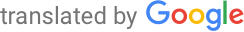Machine-translated page for increased accessibility for English questioners.

# TEI Questions

### Common basis of the program

1. Logic. Syntax, semantics and derivation systems for propositional and predicate logic, their correctness and completeness. Compactness theorem. Algorithmic decidability and complexity of satisfiability problem for propositional and predicate logic. Gödel incompleteness theorems. Resolution principle in propositional and predicate logic.
2. Probability. Discrete and continuous probability space. Random variable and its use. Mean value, variance. Random processes, Markov chains. Information theory (entropy, mutual information), coding theory (Huffman coding, capacity of error channels).
3. Complexity. Time and space computational complexity, basic complexity classes. Relation between deterministic and non-deterministic classes, Savitch theorem. Probability complexity classes. Alternative and polynomial hierarchy.
4. Database. Storage organization, addressing records. Multi-attribute hierarchical and hash indexes, bitmap indexes, dynamic hashing. Query evaluation, operation algorithms, statistics and cost estimates. Query and schema optimization, transformation rules, data splitting. Transaction Processing, Downtime and Recovery. Security, access rights.
5. Computer networks. Layer model, functionality of individual layers. Network layer protocols, IPv4 and IPv6 properties, address space. Routing: routers and their architecture, routing algorithms. Transport protocols: UDP, TCP. P2P and ad-hoc networks.

### Specialization - Algorithms of Computational Models

1. Random algorithms. Principles and methods of random algorithm creation. Probability complexity classes and their relation to deterministic complexity classes. Random walks, Markov chains and their applications. Random methods in cryptography.
2. Basics of quantum information processing. Quantum bit, quantum sources of randomness, quantum interference and superposition principle. Quantum security and uncertainty principle. Schrodinger equation and quantum gates. Quantum Bonding and Teleportation. Shor and Grover algorithm. Basic principles of quantum cryptography (one-time pad system, protocol BB84). Types of quantum measurements.
3. Numerical methods. Error analysis, absolute and relative error. Iterative methods of nonlinear equation systems, Jacobi and Gauss-Seidel methods. Solution of the system of nonlinear equations, Newton's method. Interpolation and approximation. Numerical derivation and integration.
4. Neural networks. Multilayer networks and their expressive abilities. Learning neural networks: Gradient descent, reverse propagation, practical learning issues (data preparation, weight initialization, hyperparameters selection and adaptation). Regularizace. Convolution networks. Recurrent Networks (LSTM).
5. Algorithmics for difficult problems. Algorithmic techniques for solving difficult problems: pseudopolynomial and parameterized algorithms. Approximative algorithms. Local search heuristics and genetic algorithms.

### Specialization - Formal Verification and Analysis of Programs

1. Quality concept in software development. Software metrics. Principles of Clean Code & SOLID Approaches. Code development in the development cycle. Unit testing, integration testing, system and acceptance testing. Computing performance testing and optimization. Test-based development methods. Software quality management processes. (PV260)
2. Testing methods. Testing missions and strategies, oracle problem, black-box testing and white-box testing. Code coverage. Symbolic execution as a method for error detection and test generation. (IA169)
3. Model checking. Model checking for linear and branching logic, enumerative and symbolic approach, bounded model checking. Abstraction of transition systems, CEGAR method. (IA169, IA159)
4. Programming Language Semantics. Operational, denotational and axiomatic semantics. Complete partial arrangements, fixed point theorem. While cycle denotation semantics. Assertion of partial correctness of programs, weakest input condition, invariant of the cycle, Hoare's derivation system, its correctness and completeness. Principles of automated deductive verification. (IA011)

### Specialization - Principles of Programming Languages

1. Semantics and principles of programming languages. Operational, denotational and axiomatic semantics. Complete partial arrangements, fixed point theorem. While cycle denotation semantics. Assertion of partial correctness of programs, invariant of a cycle, Hoare's derivation system. Types and type control. Declarative programming, object-oriented programming.
2. Lambda Calculus - Syntax, semantics, typed extensions (Hindley-Milner, System F), coding of data types, fixed point combiners. Modern concepts of functional programming - type classes, functors, monads and their transformers, generalized algebraic types (GADT).
3. Translators. Deterministic context-free languages and their syntactic analysis. LL (k), SLL (k), LR (k) classes and their analyzers. Semantic analysis. Names and ranges analysis, symbol table. Type control and type conversion. Code generation techniques, optimization.
4. Real time systems. The terms "soft" and "hard" real-time systems. Clock-driven and event-driven scheduling. Periodic tasks: EDF, RM and DM algorithms, optimization, scheduling tests using utilization and time-demand analysis. Aperiodic tasks: Polling server, deferrable server, sporadic server, scheduling test using time request analysis. Sporadic Tasks: Acceptance Test. Resource Planning: Priority Inversions, Deadlocks. Priority inheritance, priority ceiling protocols. Basic knowledge of multi-processor planning (time anomalies, task migration, maximum utilization, global vs partitioned planning, Dhall effect).